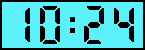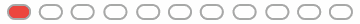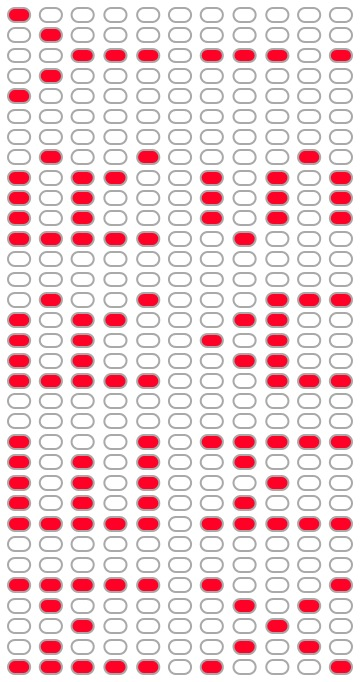## Merry Xmas!

25 Dec 2010

To everyone who participated in the Christmas Conundrum, I hope you enjoyed this little dose of holiday fun. :-)

Congrats to everyone who solved it. If you're curious, here are the first seven people who solved it, in order:

If you want the solution, keep reading.

I created the puzzle before going to bed Tuesday night. I had a lot of ideas, but I kept coming back to the idea of beginning with an animated digital clock, just like I used for a puzzle I made years ago. For that puzzle, the order of the sequence was irrelevant, so I made the clock advance pretty normally. This time, I decided I would make the sequence matter, which would make the clock seem much more sporadic. I also wanted to tailor the puzzle to an audience primarily made up of developers. Binary it is. :-)

Next, I plotted `MERRY XMAS!` in a text file:

1. `X X XXXXX XXXX XXXX X X`
2. `XX XX X X X X X X X`
3. `X X X XXXX XXXX XXXX X`
4. `X X X X X X X X`
5. `X X XXXXX X X X X X`
6. ` `
7. `X X X X X XXX X`
8. ` X X XX XX X X X X`
9. `  X X X X XXXXX XXX X`
10. ` X X X X X X X`
11. `X X X X X X XXX X`

Knowing that I wanted to use binary and wanted to use a digital clock, I knew the numbers couldn't be too big. I like creative puzzles, but I also wanted to get to bed, so I simply assigned the values vertically, like this:

1. `1024 X X XXXXX XXXX XXXX X X`
2. `512 XX XX X X X X X X X`
3. `256 X X X XXXX XXXX XXXX X`
4. `128 X X X X X X X X`
5. `64 X X XXXXX X X X X X`
6. `32`
7. `16 X X X X X XXX X`
8. `8 X X XX XX X X X X`
9. `4 X X X X XXXXX XXX X`
10. `2 X X X X X X X`
11. `1 X X X X X X XXX X`

I was still doing this by hand. (I made the old puzzle with PHP.) At this point, I regretted that decision a bit, but I powered through and quickly added up each column, from right to left:

1. `1024`
2. `512`
3. `256 + 128 + 64 + 16 + 8 + 4 + 1 = 477`
4. `512`
5. `1024`
6. ` `
7. ` `
8. `512 + 64 + 2 = 578`
9. `1024 + 256 + 128 + 16 + 4 + 1 = 1429`
10. `1024 + 256 + 16 + 4 + 1 = 1301`
11. `1024 + 256 + 16 + 4 + 1 = 1301`
12. `1024 + 512 + 256 + 128 + 64 + 8 = 1992`
13. ` `
14. ` `
15. `512 + 64 + 4 + 2 + 1 = 583`
16. `1024 + 256 + 128 + 8 + 4 = 1420 `
17. `1024 + 256 + 16 + 4 = 1300 `
18. `1024 + 256 + 8 + 4 = 1292`
19. `1024 + 512 + 256 + 128 + 64 + 4 + 2 + 1 = 1991 `
20. ` `
21. ` `
22. `1024 + 64 + 16 + 8 + 4 + 2 + 1 = 1119`
23. `1024 + 256 + 64 + 8 = 1352`
24. `1024 + 256 + 64 + 4 = 1348`
25. `1024 + 256 + 64 + 8 = 1352`
26. `1024 + 512 + 256 + 128 + 64 + 16 + 8 + 4 + 2 + 1 = 2015`
27. ` `
28. ` `
29. `1024 + 512 + 256 + 128 + 64 + 16 + 1 = 2001`
30. `512 + 8 + 2 = 522 `
31. `256 + 4 = 260`
32. `512 + 8 + 2 = 522 `
33. `1024 + 512 + 256 + 128 + 64 + 16 + 1 = 2001`

This gave me numbers small enough to work (no larger than four digits). If I had been lucky enough that each number could also represent a valid time, I was going to add one more bit of cleverness and use an analog clock with different colors to denote ante meridiem and post meridiem. But, since I wasn't so lucky, I went with the original idea of a digital clock and made this:I was pretty happy that the first two numbers made for a nice hint, and the hint I posted was really just an attempt to make it clear that these were binary numbers:Solving the puzzle required that you imagine each blink of the lights representing a horizontal slice of a larger image:Thanks again to everyone who participated. I had fun, and I hope you did, too. :-)

Merry Christmas!